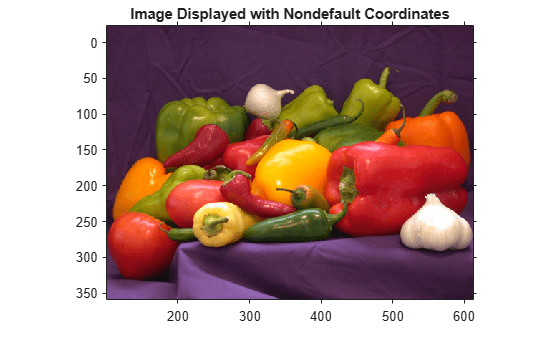Main Content

# Shift X- and Y-Coordinate Range of Displayed Image

This example shows how to specify a nondefault world coordinate system by changing the `XData` and `YData` properties of a displayed image.

Read an image.

`I = imread("peppers.png");`

Display the image using the intrinsic coordinate system, returning properties of the image in `ax`. Turn on the axis to display the coordinate system.

```figure ax = imshow(I); title('Image Displayed with Intrinsic Coordinates') axis on```Check the range of the x- and y-coordinates, which are stored in the XData and YData properties of `ax`. The ranges match the dimensions of the image.

`xrange = ax.XData`
```xrange = 1×2 1 512 ```
`yrange = ax.YData`
```yrange = 1×2 1 384 ```

Change the range of the x- and y-coordinates. This example shifts the image to the right by adding `100` to the x-coordinates and shifts the image up by subtracting `25` from the y-coordinates.

```xrangeNew = xrange + 100; yrangeNew = yrange - 25;```

Display the image, specifying the shifted spatial coordinates.

```figure axNew = imshow(I,'XData',xrangeNew,'YData',yrangeNew); title('Image Displayed with Nondefault Coordinates'); axis on```Confirm that the ranges of the x- and y-coordinates of the new image match the shifted ranges specified by `xrangeNew` and `yrangeNew`.

`axNew.XData`
```ans = 1×2 101 612 ```
`axNew.YData`
```ans = 1×2 -24 359 ```

Download ebook